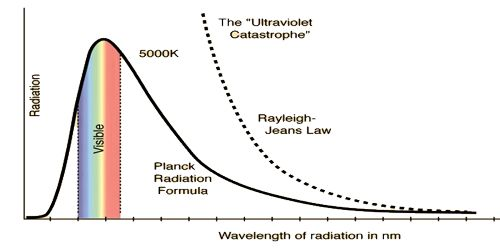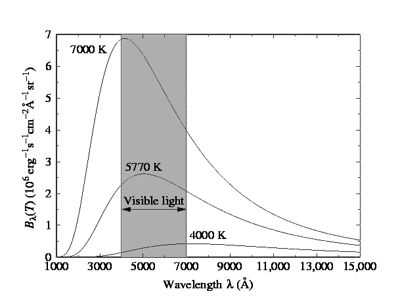Physics

# Planck’s Black Body RadiationPlanck’s Black Body Radiation

We know, radiation is emitted from a body because of temperature. Characteristics of radiation heat depending on the property of the body and its temperature. An ideal black body can absorb heat energy of all wavelengths. Again, when heated to an appropriate temperature it can emit energy of all wavelengths. An object that absorbs all radiation falling on it, at all wavelengths, is called a black body. When a black body is at a consistent temperature, its release has a feature frequency allocation that depends on the temperature. Its release is called black-body radiation. Planck’s law describes the spectral density of electromagnetic radiation emitted by a black body in thermal equilibrium at a given temperature T. In the figure, a graph has been drawn of the radiant energy versus wavelength at three different temperatures. It is seen from the graph that (1) as the temperature increases, the radiant energy also increases, and (2) the wavelength corresponding to the maximum radiant energy decreases with the increase of temperature.At a low temperature as wavelengths of all heat radiation remain in the infrared region, hence radiation cannot be seen by naked eyes. As the temperature of a body increases so light of red color slowly changes to white color. From the experimental results on heat radiation, it is seen that distribution of wavelength spectrum extends from infrared line to ultraviolet line. With increasing temperature of a black body, the emitted total energy from the blackbody also increases, but the wavelength that radiates maximum energy decreases with increasing temperature. By the theory or principle of classical mechanics, energy distribution by the black body for the whole range of spectrum cannot be explained. In order to explain black body radiation famous German scientist Max Planck in 1900 put forward a black body radiation hypothesis. After the establishment of this principle, a revolutionary era started in physics. By Wein’s energy distribution law energy radiation of low wavelengths can be explained satisfactorily. Again, by the Rayleigh and Jeans energy distribution law energy radiation at higher wavelengths can be explained. But a black body radiates low and high i.e., radiation of all wavelengths. So with the help of the above two laws black body radiation spectrum of all energy distribution cannot be explained. In order to explain energy distribution of all range scientist Planck established a theory. This theory of Planck S called quantum theory or photon theory.

According to the idea of Planck energy radiation from a body or exchange of energies between different bodies do not take place continuously. There is no continuity in this process. Emission or absorption of energies occurs discontinuously and discretely, always as integral multiples of energy unit of energy or packet or bundle of energy which Planck called quantum. Each emery particle or quanta of energy is an

integral unit. This integral unit of energy is called quantum or photon. This quantum or photon is called atoms of energy. If the frequency of the quantum or photon is v and Planck’s constant is ‘h’, then energy in a photon is,

E = hv

But if n-number of photons are emitted or absorbed at a time, then total energy = nhv, here n = 0, 1, 2, … , etc. This is the Planck’s radiation law. Planck constant, h = 6.63 x 10-27 erg-sec. This theory of radiation is known as quantum theory.